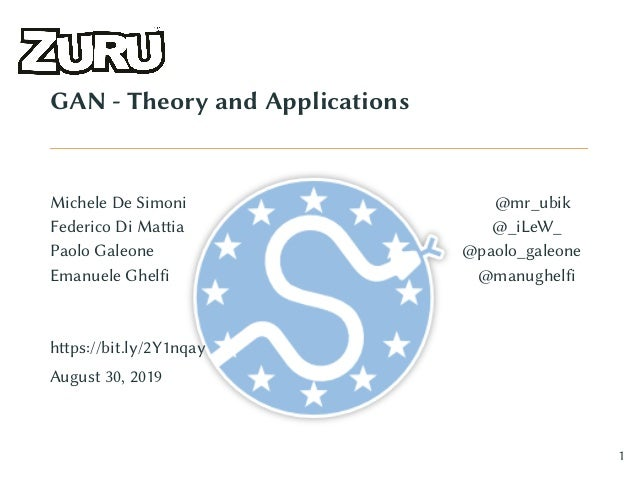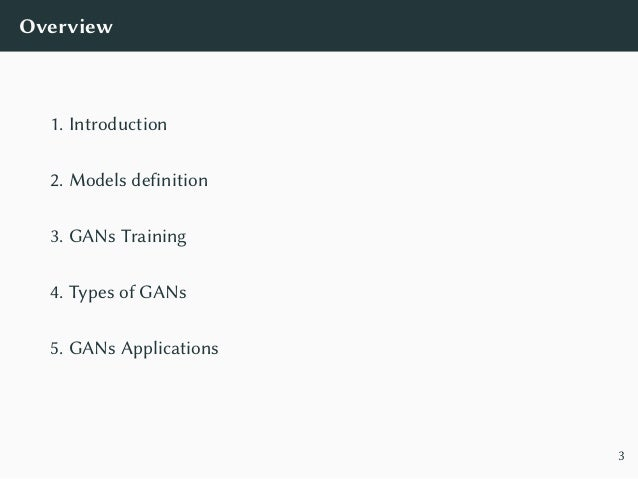Successfully reported this slideshow.Upcoming SlideShare
×

ofUpcoming SlideShare
Next

2 Likes

Share

# EuroSciPy 2019 - GANs: Theory and Applications

EuroSciPy 2019: https://pretalx.com/euroscipy-2019/talk/Q79NND/

GANs are the new hottest topic in the ML arena; however, they present a challenge for the researchers and the engineers alike. Their design, and most importantly, the code implementation has been causing headaches to the ML practitioners, especially when moving to production.

The workshop aims at providing a complete understanding of both the theory and the practical know-how to code and deploy this family of models in production. By the end of it, the attendees should be able to apply the concepts learned to other models without any issues.

We will be showcasing all the shiny new APIs introduced by TensorFlow 2.0 by showing how to build a GAN from scratch and how to "productionize" it by leveraging the AshPy Python package that allows to easily design, prototype, train and export Machine Learning models defined in TensorFlow 2.0.

The workshop is composed of:

- Theoretical introduction
- GANs from Scratch in TensorFlow 2.0
- High-performance input data pipeline with TensorFlow Datasets
- Introduction to the AshPy API
- Implementing, training, and visualizing DCGAN using AshPy
- Serving TF2 Models with Google Cloud Functions
The materials of the workshop will be openly provided via GitHub (https://github.com/zurutech/gans-from-theory-to-production).

See all

See all

### EuroSciPy 2019 - GANs: Theory and Applications

1. 1. GAN - Theory and Applications Michele De Simoni @mr_ubik Federico Di Mattia @_iLeW_ Paolo Galeone @paolo_galeone Emanuele Ghelfi @manughelfi https://bit.ly/2Y1nqay August 30, 2019 1
2. 2. Overview 1. Introduction 2. Models definition 3. GANs Training 4. Types of GANs 5. GANs Applications 3
3. 3. Introduction
4. 4. “ Generative Adversarial Networks is the most interesting idea in the last ten years in machine learning. Yann LeCun, Director, Facebook AI ” 4
5. 5. Generative Adversarial Networks Two components, the generator and the discriminator: • The generator G needs to capture the data distribution. • The discriminator D estimates the probability that a sample comes from the training data rather than from G. Figure 1: Credits: Silva 5
6. 6. Generative Adversarial Networks GANs game: min G max D VGAN(D, G) = E x∼pdata(x) [log D(x)]+ E z∼pz (z) [log(1−D(G(z)))] 6
7. 7. Generative Adversarial Networks GANs game: min G max D VGAN(D, G) = E x∼pdata(x) [log D(x)] real samples + E z∼pz (z) [log(1−D(G(z)))] 6
8. 8. Generative Adversarial Networks GANs game: min G max D VGAN(D, G) = E x∼pdata(x) [log D(x)] real samples + E z∼pz (z) [log(1 − D(G(z)))] generated samples 6
9. 9. GANs - Discriminator • Discriminator needs to: • Correctly classify real data: max D E x∼pdata(x) [log D(x)] D(x) → 1 • Correctly classify wrong data: max D E z∼pz (z) [log(1 − D(G(z)))] D(G(z)) → 0 • The discriminator is an adaptive loss function. 7
10. 10. GANs - Generator • Generator needs to fool the discriminator: • Generate samples similar to the real ones: min G E z∼pz (z) [log(1 − D(G(z)))] D(G(z)) → 1 9
11. 11. GANs - Generator • Generator needs to fool the discriminator: • Generate samples similar to the real ones: min G E z∼pz (z) [log(1 − D(G(z)))] D(G(z)) → 1 • Non saturating objective (Goodfellow et al., 2014): min G E z∼pz (z) [− log(D(G(z)))] 9
12. 12. GANs - Generator Objectives • Minimax: log(1 − D(G(z))) 0 0.5 1 −6 −4 −2 0 2 4 D(G(z)) JG Minimax 10
13. 13. GANs - Generator Objectives • Minimax: log(1 − D(G(z))) • Non-saturating: − log(D(G(z))) 0 0.5 1 −6 −4 −2 0 2 4 D(G(z)) JG Minimax Non-saturating 10
14. 14. Models definition
15. 15. GANs - Models definition • Different architectures for different data types. • Tuple of numbers? Fully Connected Neural Networks 11
16. 16. GANs - Models definition • Different architectures for different data types. • Text or sequences? Recurrent Neural Networks 11
17. 17. GANs - Models definition • Different architectures for different data types. • Images? Convolutional Neural Networks 1 latent 1 fc 32768 256 *conv1 32 128 *conv2 64 64 *conv3 128 K *conv4 128 3 128 conv5 Latent Vector Conv/Deconv Fully Connected Batch Norm Relu 11
18. 18. GANs Training
19. 19. GANs - Training • D and G are competing against each other. • Alternating execution of training steps. • Use minibatch stochastic gradient descent/ascent. 12
20. 20. GANs - Training - Discriminator How to train the discriminator? Repeat from 1 to k: 1. Sample minibatch of m noise samples z(1), . . . , z(m) from pz(z) 13
21. 21. GANs - Training - Discriminator How to train the discriminator? Repeat from 1 to k: 1. Sample minibatch of m noise samples z(1), . . . , z(m) from pz(z) 2. Sample minibatch of m examples x(1), . . . , x(m) from pdata(x) 13
22. 22. GANs - Training - Discriminator How to train the discriminator? Repeat from 1 to k: 1. Sample minibatch of m noise samples z(1), . . . , z(m) from pz(z) 2. Sample minibatch of m examples x(1), . . . , x(m) from pdata(x) 3. Update D: J = 1 m m i=1 log D(x(i) ) + log(1 − D(G(z(i) ))) D performance θd = θd + λ θd J 13
23. 23. GANs - Training - Generator How to train the generator? Update executed only once after D updates: 1. Sample minibatch of m noise samples z(1), . . . , z(m) from pz(z) 14
24. 24. GANs - Training - Generator How to train the generator? Update executed only once after D updates: 1. Sample minibatch of m noise samples z(1), . . . , z(m) from pz(z) 2. Update G: J = 1 m m i=1 log(D(G(z(i) ))) G performance θg = θg + λ θgJ 14
25. 25. GANs - Training - Considerations • Optimizers: Adam, Momentum, RMSProp. • Arbitrary number of steps or epochs. • Training is completed when D is completely fooled by G. • Goal: reach a Nash Equilibrium where the best D can do is random guessing. 15
26. 26. Types of GANs
27. 27. Types of GANs Two big families: • Unconditional GANs (just described). • Conditional GANs (Mirza and Osindero, 2014). 16
28. 28. Conditional GANs • Both G and D are conditioned on some extra information y. • In practice: perform conditioning by feeding y into D and G. Figure 2: From Mirza and Osindero (2014) 17
29. 29. Conditional GANs The GANs game becomes: min G max D E x∼pdata(x|y) [log D(x, y)] + E z∼pz (z) [log(1 − D(G(z|y), y))] Notice: the same representation of the condition has to be presented to both network. 18
30. 30. GANs Applications
31. 31. Unconditional - Face Generation - Karras et al. (2017) 19
32. 32. Conditional - Domain Translation - Isola et al. (2016) 20
33. 33. Conditional - Semantic Image Synthesis - Park et al. (2018) 21
34. 34. Conditional - Image Super Resolution - Ledig et al. (2016) 22
35. 35. Real-world GANs • Semi-Supervised Learning (Salimans et al., 2016) • Image Generation (almost all GAN papers) • Image Captioning • Anomalies Detection (Zenati et al., 2018) • Program Synthesis (Ganin et al., 2018) • Genomics and Proteomics (Killoran et al., 2017) (De Cao and Kipf, 2018) • Personalized GANufactoring (Hwang et al., 2018) • Planning
36. 36. References [De Cao and Kipf 2018] De Cao, Nicola ; Kipf, Thomas: MolGAN: An Implicit Generative Model for Small Molecular Graphs. (2018). – (2018) [Ganin et al. 2018] Ganin, Yaroslav ; Kulkarni, Tejas ; Babuschkin, Igor ; Eslami, S. M. A. ; Vinyals, Oriol: Synthesizing Programs for Images Using Reinforced Adversarial Learning. (2018). – (2018) [Goodfellow et al. 2014] Goodfellow, Ian J. ; Pouget-Abadie, Jean ; Mirza, Mehdi ; Xu, Bing ; Warde-Farley, David ; Ozair, Sherjil ; Courville, Aaron ; Bengio, Yoshua: Generative Adversarial Networks. (2014). – (2014)
37. 37. [Hwang et al. 2018] Hwang, Jyh-Jing ; Azernikov, Sergei ; Efros, Alexei A. ; Yu, Stella X.: Learning Beyond Human Expertise with Generative Models for Dental Restorations. (2018). – (2018) [Isola et al. 2016] Isola, Phillip ; Zhu, Jun-Yan ; Zhou, Tinghui ; Efros, Alexei A.: Image-to-Image Translation with Conditional Adversarial Networks. (2016). – (2016) [Karras et al. 2017] Karras, Tero ; Aila, Timo ; Laine, Samuli ; Lehtinen, Jaakko: Progressive Growing of GANs for Improved Quality, Stability, and Variation. (2017). – (2017) [Killoran et al. 2017] Killoran, Nathan ; Lee, Leo J. ; Delong, Andrew ; Duvenaud, David ; Frey, Brendan J.: Generating and Designing DNA with Deep Generative Models. (2017). – (2017)
38. 38. [Ledig et al. 2016] Ledig, Christian ; Theis, Lucas ; Huszar, Ferenc ; Caballero, Jose ; Cunningham, Andrew ; Acosta, Alejandro ; Aitken, Andrew ; Tejani, Alykhan ; Totz, Johannes ; Wang, Zehan ; Shi, Wenzhe: Photo-Realistic Single Image Super-Resolution Using a Generative Adversarial Network. (2016). – (2016) [Mirza and Osindero 2014] Mirza, Mehdi ; Osindero, Simon: Conditional Generative Adversarial Nets. (2014). – (2014) [Park et al. 2018] Park, Taesung ; Liu, Ming-Yu ; Wang, Ting-Chun ; Zhu, Jun-Yan: Semantic Image Synthesis with Spatially-Adaptive Normalization. (2018). – (2018) [Salimans et al. 2016] Salimans, Tim ; Goodfellow, Ian ; Zaremba, Wojciech ; Cheung, Vicki ; Radford, Alec ; Chen, Xi: Improved Techniques for Training GANs. (2016). – (2016)
39. 39. [Silva ] Silva, Thalles: An Intuitive Introduction to Generative Adversarial Networks (GANs) [Zenati et al. 2018] Zenati, Houssam ; Foo, Chuan S. ; Lecouat, Bruno ; Manek, Gaurav ; Chandrasekhar, Vijay R.: Efficient GAN-Based Anomaly Detection. (2018). – (2018)
•#### MichelleOlson48

Nov. 26, 2021
•Feb. 5, 2021

Total views

945

On Slideshare

0

From embeds

0

Number of embeds

4

38

Shares

0